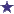# How Many MLs of Diluent Needed in this Question?

by Joe
(New York)Dilution of 50 mg/ml and Stock Solution of 2 gm/5ml

You have been asked to prepare 20 mL of a drug dilution of 50 mg/mL from a stock solution of 2 g/5 mL. How many milliliters of diluent will be needed?

### Comments for How Many MLs of Diluent Needed in this Question?

Average RatingRatingby: David So the stock solution is 2 grams per 5mL. 2gm/5mL is equal to 2000mg/5mL, which is also equal to 400mg/ml. The ratio we want to end up with is 50mg/1ml. The following ratios are the same as that: 100mg/2mL, 200mg/4mL, and 250mg/5mL. 400mg/mL is more concentrated by a factor of 8 than 50mg/mL. How many milliliters of diluent will be needed to prepare 20ml of the drug dilution? Now, the stock solution is 8 times more concentrated than the ratio we want to end up with (50mg/mL). 1/8 = 0.125 ...